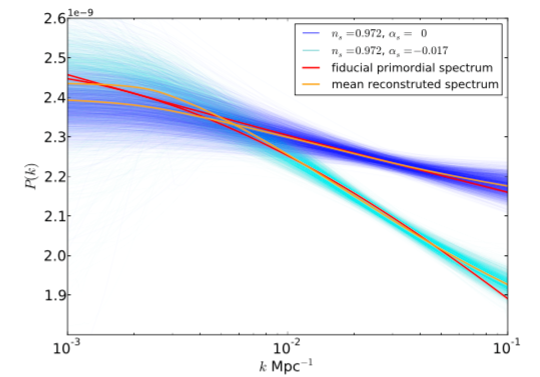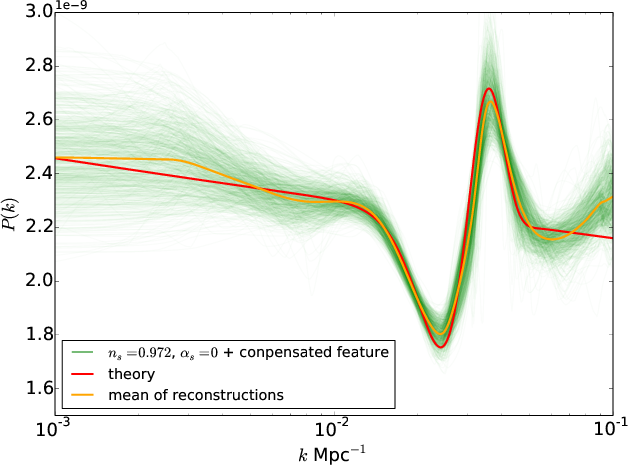# PRISM: Sparse Recovery of the Primordial Power SpectrumAuthors: P. Paykari, F. Lanusse, J. -L. Starck et al. Journal: A&A Year: 2014 Download: ADS | arXiv

## Abstract

Aims. The primordial power spectrum describes the initial perturbations in the Universe which eventually grew into the large-scale structure we observe today, and thereby provides an indirect probe of inflation or other structure-formation mechanisms. Here, we introduce a new method to estimate this spectrum from the empirical power spectrum of cosmic microwave background (CMB) maps.
Methods. A sparsity-based linear inversion method, coined PRISM, is presented. This technique leverages a sparsity prior on features in the primordial power spectrum in a wavelet basis to regularise the inverse problem. This non- parametric approach does not assume a strong prior on the shape of the primordial power spectrum, yet is able to correctly reconstruct its global shape as well as localised features. These advantages make this method robust for detecting deviations from the currently favoured scale-invariant spectrum.
Results. We investigate the strength of this method on a set of WMAP 9-year simulated data for three types of primordial power spectra: a nearly scale-invariant spectrum, a spectrum with a small running of the spectral index, and a spectrum with a localised feature. This technique proves to easily detect deviations from a pure scale-invariant power spectrum and is suitable for distinguishing between simple models of the inflation. We process the WMAP 9-year data and find no significant departure from a nearly scale-invariant power spectrum with the spectral index ns = 0.972. Conclusions. A high resolution primordial power spectrum can be reconstructed with this technique, where any strong local deviations or small global deviations from a pure scale-invariant spectrum can easily be detected.

## Summary

The primordial power spectrum describes the initial perturbations in the Universe which eventually grew into the large-scale structure we observe today, and thereby provides an indirect probe of inflation or other structure-formation mechanisms. The primordial power spectrum is therefore linked to cosmological observables and in particular the Cosmic Microwave Background (CMB). However, due to degeneracies in the transfer function that links the primordial power spectrum to the measured CMB, recovering the primordial power spectrum constitutes a non-trivial ill-posed inverse problem. Furthermore, the multiplicative nature of the noise on the estimator of CMB power spectrum adds to the complexity of the problem. PRISM is a new sparsity-based, non-linear reconstruction technique which aims at reconstructing global and local features on the primordial power spectrum from CMB measurements. Features are detected and reconstructed based on their significance compared to noise. The PRISM software is part of the ISAP package and will be included in the next release of the package. The figure below shows reconstructions of a primordial power spectrum with a localized feature around 0.03 Mpc using PRISM.## The algorithm

The primordial power spectrum reconstruction problem is an ill-posed linear inverse problem which we address through the framework of sparse regularisation. This framework allows for accurate and robust recovery by leveraging a sparsity prior on the signal to recover (i.e. it is assumed that it can be represented using a small number of coefficients in an adapted dictionary). Formally, the observed pseudo-power spectrum $$\widetilde{C}_\ell$$ computed from masked CMB maps can be linked to the underlying primordial power spectrum $$P_k$$ through a linear relation of the form: $\widetilde{C}_\ell = \left( \mathbf{M} P_k \right) Z_\ell$ where $$\mathbf{M}$$ is a linear operator that encodes the angular transfer function of CMB anisotropies and the effects of masks and beams, and $$\mathbf{Z_\ell}$$ is a multipicative noise term. The recovery of the primordial power spectrum is performed by solving an optimization problem of the form: $\min\limits_{X} \quad \frac{1}{2} \underbrace{\parallel C_\ell - \mathbf{M} X \parallel^{2}_{2}}_{\mbox{data fidelity term}} \quad + \quad \lambda \underbrace{\parallel \mathbf{\Phi} X \parallel_{1}}_{\mbox{sparsity promoting term}}$ where $$\mathbf{\Phi}$$ is a wavelet transform. To estimate the unknown quantity $$C_\ell - \mathbf{M} X$$ from the noisy quantity $$\widetilde{C}_\ell - \mathbf{M} X$$, we use a variance stabilisation approach derived from the technique used in the TOUSI algorithm (Paykari et al. (2012)) and based on the Whaba variance stabilisation transform.

## Results on simulations

To assess the performance of PRISM we produced a large number of Monte-Carlo CMB simulations for different test power spectra. We tested a near scale invariant primordial power spectra with ns=0.972, a power spectrum with a running of the spectral index with ns=0.972, $$\alpha_s$$= -0.017 (see figure below), and a power law spectrum with a localised feature around 0.3 Mpc (see above figure).